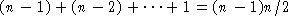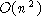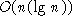# Sorting

Important task is to be able to sort information--lists of names, numbers, etc.

Again can have internal (in memory) and external (data kept in external files) sorts. Will only look at internal sorts.

Knuth defines 25 internal sorting algorithms. We will look at a few.

## Performance

As in searching a key measure of performance is the number of comparisons. In addition, for sorting algorithms, we also look at the amount of data movement (swaps, shifting of data).

We will use array implementations for demonstrating the algorithms. Some of the algorithms are also suitable for linked lists.

## Driver Program

```/*
* /cs/cs2005/pub/example/sort.C
*
* usage: sort [-t] sorttype
*/

#include <iostream.h>

typedef short BOOLEAN;
#define TRUE 1
#define FALSE 0

#define MAXINT 100

void Trace(int [], int , int , BOOLEAN );
void Swap(int [], int, int);
void BubbleSort(int [], int, BOOLEAN);
void SelectionSort(int [], int, BOOLEAN);
void MergeSort(int [], int, int);
void Merge(int [], int, int, int);
void QuickSort(int [], int, int);
int Partition(int [], int, int);

main(int argc, char *argv[])
{
int rgInt[MAXINT], cnt, i;
char *sbType;                /* type of sort */
BOOLEAN bTrace;

cnt = 0;
bTrace = FALSE;

/* process command line arguments */
if (argc == 1) {
cout << "No sort type given\n";
exit(1);                /* no type given */
}
else if (strcmp(argv, "-t") == 0) {
bTrace = TRUE;
if (argc >= 3)
sbType = argv;
else
exit(1);                /* no type given */
}
else
sbType = argv;

/* fill up the array from input */
while ((cin >> rgInt[cnt]) && !cin.eof())
cnt++;

if (strcmp(sbType, "bubble") == 0)
BubbleSort(rgInt, cnt, bTrace);
else if (strcmp(sbType, "selection") == 0)
SelectionSort(rgInt, cnt, bTrace);
else if (strcmp(sbType, "merge") == 0)
MergeSort(rgInt, 0, cnt-1);
else if (strcmp(sbType, "quick") == 0)
QuickSort(rgInt, 0, cnt-1);
else {
cout << "Unknown sort type: "<< sbType << "\n";
exit(1);
}
cout << "Ordered list:";
for (i = 0; i < cnt; i++)
cout << " " <<  rgInt[i];
cout << "\n";
}```

```/*
* Trace -- print optional trace info
*/
void Trace(int rgInt[], int cnt, int i, BOOLEAN bTrace)
{
int k;

if (bTrace) {
cout << "Trace i = " << i << ":";
for (k = 0; k < cnt; k++)
cout << " " << rgInt[k];
cout << "\n";
}
}

/*
* Swap -- swap two elements of the array
*/
void Swap(int rgInt[], int i, int j)
{
int tmp;

tmp = rgInt[i];
rgInt[i] = rgInt[j];
rgInt[j] = tmp;
}```

## Bubble Sort

For each pass through the array, ``bubble'' the largest value to the right. Use n-1 passes to ensure that all elements are sorted.

```/*
* BubbleSort -- use bubblesort to sort an array
*/
void BubbleSort(int rgInt[], int cnt, BOOLEAN bTrace)
{
int i, j;

for (i = cnt-1; i > 0; i--) {
for (j = 0; j < i; j++)
if (rgInt[j] > rgInt[j+1])
Swap(rgInt, j, j+1);
Trace(rgInt, cnt, i, bTrace);
}
}```

### Sample Execution

```> sort -t bubble
4 6 1 2 7 8 3
Trace i =  6:  4  1  2  6  7  3  8
Trace i =  5:  1  2  4  6  3  7  8
Trace i =  4:  1  2  4  3  6  7  8
Trace i =  3:  1  2  3  4  6  7  8
Trace i =  2:  1  2  3  4  6  7  8
Trace i =  1:  1  2  3  4  6  7  8
Ordered list:  1  2  3  4  6  7  8```

### Analysis

Number of comparisons: thus bubble sort is.

Number of swaps: in the worst case will be the same if list is in reverse sorted order

## Selection Sort

For each pass through the array, select the highest value and swap its location with the end of the list. Again use n-1 passes to ensure that all elements are sorted. However it involves less data movement.

```/*
* MaxKey -- return the index of the maximum value in the range
*/
int MaxKey(int rgInt[], int low, int high)
{
int i, max;

max = low;
for (i = low + 1; i <= high; i++)
if (rgInt[max] < rgInt[i])
max = i;
return(max);
}

/*
* SelectionSort -- use selectionsort to sort an array
*/
void SelectionSort(int rgInt[], int cnt, BOOLEAN bTrace)
{
int i, j;

for (i = cnt-1; i > 0; i--) {
j = MaxKey(rgInt, 0, i);
Swap(rgInt, i, j);
Trace(rgInt, cnt, i, bTrace);
}
}```

### Sample Execution

```> sort -t selection
4 6 1 2 7 8 3
Trace i =  6:  4  6  1  2  7  3  8
Trace i =  5:  4  6  1  2  3  7  8
Trace i =  4:  4  3  1  2  6  7  8
Trace i =  3:  2  3  1  4  6  7  8
Trace i =  2:  2  1  3  4  6  7  8
Trace i =  1:  1  2  3  4  6  7  8
Ordered list:  1  2  3  4  6  7  8```

### Analysis

Number of comparisons: thus selection sort is.

Number of swaps: will be one swap on each loop so it is O(n).

## Better Approaches

We can prove that the lower bound on sorting is actually .

The approach for reaching this lower bound is to use divide and conquer. Sorting is easier on short lists than long list. For example in previous algorithms we havebehavior.

Thus general approach is:

```Sort(list)
{
if (list length greater than 1) {
Partition the list into lowlist, highlist;
Sort(lowlist);
Sort(highlist);
Combine(lowlist, highlist);
}
}```

Natural use of recursion.

Key question is how to partition the two lists.

Will look at two approaches.

## Merge Sort

At each point simply divide the list into two and sort each list. Trivial (or base) case is when the sublist has 0 or 1 elements.

```/* MergeSort --
* Programmer: David Finkel
* Parameters: array to be sorted, low and high limits of indices to
*             be sorted
*/
void MergeSort(int rgInt[], int low, int high)
{
int middle;

if (low >= high)                /* if list is empty or has only one element */
return;
else {
middle = (low + high) / 2;
MergeSort(rgInt, low, middle);
MergeSort(rgInt, middle + 1, high);
Merge(rgInt, low, middle, high);
}
}```

```/* Merge --
* Programmer: David Finkel
* merges two sorted portions of the rgInt array, the first from low
* to middle, and the second from middle + 1 to high
*/
void Merge(int rgInt[], int low, int middle, int high)
{
int first, second;    /* indices into the two portions of the array */
int rgTemp[MAXINT];    /* array to hold sorted elements  */
int third;            /* index into rgTemp array */
int i;                /* loop index */

first = low;
second = middle + 1;
third = low;

while ((first <= middle) && (second <= high)) { /* while sub-lists are
not exhausted */
if ( rgInt[first] <= rgInt[second] ) { /* copy smaller elt into rgTemp */
rgTemp[third] = rgInt[first];
first++;
}
else {
rgTemp[third] = rgInt[second];
second++;
}
third++;
}
/* copy remainder of non-exhausted sub-list into rgTemp */
if (first > middle)          /* first sub-list is exhausted */
while ( second <= high ) {
rgTemp[third] = rgInt[second];
second++;
third++;
}
else                         /* second sub-list is exhausted */
while ( first <= middle ) {
rgTemp[third] = rgInt[first];
first++;
third++;
}

/* copy rgTemp back over original rgInt array */
for ( i = low; i <= high; i++)
rgInt[i] = rgTemp[i];

return;
}  /* end of merge */```

```> sort merge
4 6 1 2 7 8 3
Ordered list:  1  2  3  4  6  7  8```

### Analysis

Comparisons are only done in the Merge() routine as it combines two lists. We can have no more comparisons than elements being merged. Hence if we look at Figure 7.10 we see a total of n elements at each level andlevels for a total of . Thus merge sort isin number of comparisons.

Biggest disadvantage is that it requires an auxilliary array when using array storage. Would not need this extra storage with a linked list implementation (done in Kruse text).

## Quick Sort

Use a pivot to divide the list. Do not pick the first element as the pivot in case the list is already (partially) sorted.

Pick a pivot in the middle of the list and work through the algorithm shown on pg 248 of Kruse text.

Quick Sort is done recursively.

```/* QuickSort --
* Programmer: David Finkel
* parameters: array to be sorted, low and high limits of indices to
*             be sorted
*/
void QuickSort(int rgInt[], int low, int high)
{
int pivot_loc;

if ( low >= high )       /* if only one element to be sorted  */
return;
else {
pivot_loc = Partition(rgInt, low, high);
QuickSort(rgInt, low, pivot_loc - 1);
QuickSort(rgInt, pivot_loc + 1, high);
}
} /* end of merge_sort */```

```/* partition function
* parameters: array to be sorted, low and high indices to be sorted
* returns: pivot location
*/
int Partition(int rgInt[], int low, int high)
{
int i, pivot_loc, pivot_val;

Swap(rgInt, low, (low + high)/2);
pivot_val = rgInt[low];               /* select a pivot */
pivot_loc = low;                     /* record pivot's location */
for (i = low + 1; i <= high; i++)
if (rgInt[i] < pivot_val)
Swap(rgInt, ++pivot_loc, i);   /* found an entry smaller than pivot */
Swap(rgInt, low, pivot_loc);
return(pivot_loc);
} /* end partition */```

```> sort quick
4 6 1 2 7 8 3
Ordered list:  1  2  3  4  6  7  8```

### Analysis

Worst case number of comparisons (if we pick a bad pivot each time that does not divide the list) isbehavior.swaps in worst case.

However, on average the worst case does not happen and the average case isand unlike merge sort it requires no auxillary array.

## Comparison of Methods

Criteria:

• Comparisons (most often used basis of comparison)

• Data Movement (selection sort is good)